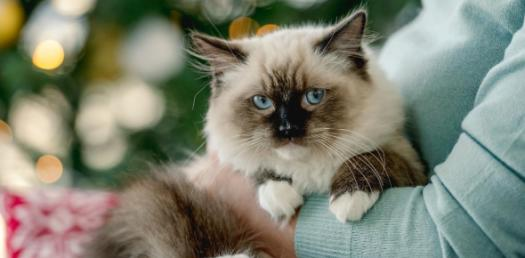# Cat - Numbers And Systems - 001

10 Questions | Attempts: 114
ShareSettingsThis is a test on Numbers for the CAT Examination.

• 1.
What is the number of factors of 70560?
• A.

15

• B.

108

• C.

20

• D.

216

• E.

5

• 2.
Find the total number of sets of factors of 264 which are co-prime to each other?
• A.

11

• B.

1

• C.

9

• D.

13

• E.

31

• 3.
How many numbers lesser than 72 are co-prime to it?
• A.

24

• B.

12

• C.

8

• D.

3

• E.

16

• 4.
How many factors of 7200 are odd?
• A.

9

• B.

2

• C.

4

• D.

10

• E.

None of these

• 5.
Find the total number of factors of 104 divisible by 13?
• A.

3

• B.

4

• C.

5

• D.

6

• E.

8

• 6.
How many factors of 5625 are not perfect squares?
• A.

10

• B.

9

• C.

12

• D.

6

• E.

5

• 7.
Find the sum of co-primes less than 14?
• A.

50

• B.

63

• C.

42

• D.

35

• E.

25

• 8.
3454565X4 is divisible by 8. Find X?
• A.

0 or 8

• B.

4 or 8

• C.

2 or 4 or 8

• D.

0 or 4 or 8

• E.

0 or 2 or 4

• 9.
In how many ways can 91091 be written as a product of two co-primes?
• A.

18

• B.

8

• C.

4

• D.

16

• E.

None of these

• 10.
Find the sum of the factors of 360?
• A.

650

• B.

780

• C.

1040

• D.

985

• E.

1170

## Related TopicsBack to top
×

Wait!
Here's an interesting quiz for you.